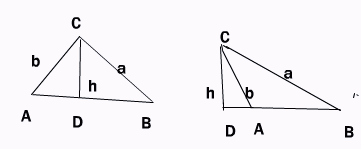Email us to get an instant 20% discount on highly effective K-12 Math & English kwizNET Programs!

#### Online Quiz (WorksheetABCD)

Questions Per Quiz = 2 4 6 8 10

### Geometry8.7 Relation Between the Sides of a Triangle and T-Ratios

 The Law of sines In any triangle, the sides are proportional to the sines of the opposite angles, i.e., a/sin A = b/sin B = c.sin C Proof: Let ABC be any oblique triangle. Draw the altitude CD = h. Two cases are possible; all angles are acute or one angle obtuse. In either case; Sin B = h/a from the first figure h = a sin B From the second figure sin A = h/b h = b sin AEquating the two values of h we get, asin B = bsin A => a/sin A = b/sin B Similarly by drawing perpendicular from A to BC, we can prove that c/sin C = b/sin B Therefore in any triangle a/sin A = b/sin B = c/sin C Relations between three sides and two angles In any triangle, to prove that a = b cos C + c cos B, b = c cos A + a cos C, c = a cos B + b cos A In the first figure we have AD/AC = cos A , hence AD = AC cos A = b cos A DB/BC = cos B, hence DB = BC cos B = a cos B Now, AB = AD + DB = b cos A + a cos B => c = a cos B + b cos A Similarly, we can prove the other relations. The law of cosines The square on any side of a triangle is equal to the sum of the squares on the other two sides minus twice the product of those two sides and the cosine of the included angle, i.e, a2 = b2 + c2 - 2bc cos A b2 = c2 + a2 - 2ca cos B c2 = a2 + b2 - 2ab cos C Proof: Consider any oblique triangle ABC with the altitude CD drawn from the vertex C to the opposite side.From the first figure we have AD = b cos A, CD = b sin A, DB = AB - AD = c-b cos A From figure 2 we have cos A = cos (180 - ang CAD) = -cos CAD = - AD/b => AD = -b cos A; CD = b sin A; DB = DA + AB = c - b cos A Thus in both figures, DB = c - b cos A, CD = b sin A Hence a2 = DB2 + CD2 (Pythagoras theorem) = (c-b cos A)2 + b2 sin 2A => a2 = c2 - 2bc cos A + b2(cos2A + b2Sin2A). Hence a2 = b2 + c2 - 2bc cos A Other results can be proved similarly. Cos A = b2 + c2 - a2/2bc Cos B = c2 + a2 - b2/2ca Cos C = a2 + b2 - c2/2ab Area of a triangle A = s(s-a)(s-b)(s-c)1/2 s = (a+b+c)/2 where a, b and c are sides of the triangle . Directions: Solve the following.
 Q 1: In triangle ABC b = 4, c = 6, B = 30o, find sin C.3/41/24/3 Q 2: In triangle ABC, if b = 6, c = 4, cos A = 1/12, find a431/24.31/2 Q 3: In triangle ABC, a = 50, c = 60, b = 100 degrees, find b.8084.585 Q 4: In a triangle ABC, a = 2, b = 3 and sin A = 2/3, find angle B.75 degrees80 degrees90 degrees Q 5: In triangle ABC, a= 15 cm, B = 68 degrees, C = 43 degrees, find b, c and B.A = 60 degrees, b = 4 cm, c = 9 cmA = 60 degrees, b = 3 cm, c = 7 cmA = 69 degrees, b = 4.89 cm, c = 10.96 cm Q 6: The angles of a triangle ABC are in AP and b:c = 31/2:21/2. Find angle A.60 degrees75 degrees45 degrees Q 7: Solve the triangle ABC given that A = 67o, b = 3 cms, c = 2 cms.a = 2.88 cm, B = 73o19l, C = 39o 41la = 2 cm, B = 73oC = 39o 41la = 5 cm, B = 70o19l, C = 39o 41l Q 8: In triangle ABC, a = 8.4 cm, b = 7.6 cm and c = 9.2 cm. Calculate the area of triangle ABC.30 sq cm45 sq cm50 sq. cm Question 9: This question is available to subscribers only! Question 10: This question is available to subscribers only!